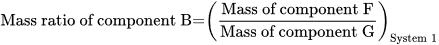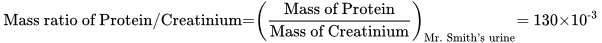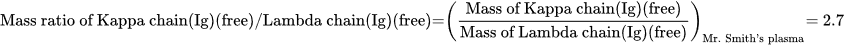Select Page

# Mass ratio

### Definition in words

The mass of component F divided by mass of component G in system 1

Note 1: Component F ≠ Component G (≠ = “not equal to”)

Note 2: Component F ∉ Component G (∉ = “is not an element of”)

Note 3: The quotient of mass of component F and mass of component G is denoted “mass ratio of component B”

### Definition by equation### Systematic terms

Mass ratio of component B in system 1

### Result type

Ratio scale
• Value sets are positive numerical values
• Algebraic operations are allowed (a=nb; a≠nb)
Numerical values from 0-∞

### Unit

10-6, 10-3, 1 (one), 103, 106, etc.

Unit, one (1) is omitted in the NPU syntax

Weight ratio

### Reference

Ferard G, Dybkaer R, Fuentes-Arderiu X. Compendium of Terminology and Nomenclature of Properties in Clinical Laboratory Sciences : Recommendations 2016. 1 ed: Royal Society of Chemistry; 2016. 182 p.

### Clinical Chemistry

Example 1
Generic abbreviated
NPU27314 U—Protein/Creatininium; mass ratio = ? × 10-3
Full result NPU27314 Urine—Protein/Creatininium; mass ratio = 130 × 10-3
Abbreviated result NPU27314 U—Protein/Creatininium; mass ratio = 130 × 10-3
Written expression The mass ratio of Protein/Creatininium in Mr. Smith’s urine is  × 10-3.
EquationNotes

The unit “10-3” represents various expressions for the same unit and magnitude:

Example 2
Generic abbreviated
NPU19608 P—Kappa chain(Ig)(free)/Lambda chain(Ig)(free); mass ratio = ?
Full result NPU19608 Plasma—Kappa chain(Ig)(free)/Lambda chain(Ig)(free); mass ratio = 2.7 times one
Abbreviated result NPU19608 P—Kappa chain(Ig)(free)/Lambda chain(Ig)(free); mass ratio = 2.7
Written expression The mass ratio of Kappa chain(Ig)(free)/Lambda chain(Ig)(free) in Mr. Smith’s plasma is [2.7].
EquationNotes The unit “1” represents various expressions for the same unit and magnitude,

### Reference

NPU database, https://www.ifcc.org/ifcc-scientific-division/sd-committees/c-npu/npusearch/ and http://www.labterm.dk/default.aspx
• # Kind-of-property

• ## Kind-of-quantity

### Reference

• Dybkaer R. Concept system on ‘quantity’: formation and terminology. Accredit Qual Assur. 2013;18(3):253-60.
• Dybkaer R. ISO terminological analysis of the VIM3 concepts ‘quantity’ and ‘kind-of-quantity’. Metrologia. 2010;47(3):127-34.
Date Term Note
1996-01-01 Mass rate Term established

QU50134